# 线性代数-矩阵与图形

## 1从行列式和多项式开始

1. 不同行列的所有排列数元素相乘根据逆序数变号，后加总。 $\det (A) = \sum\limits_{\sigma \in {S_n}} {{\mathop{\rm sgn}} (\sigma )\prod\limits_{i = 1}^n {{a_{i,\sigma (i)}}} }$
2. 代数余子式分解计算。

### 1.1行列式与空间图形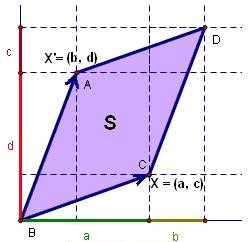1. 当 ${\alpha _1},{\alpha _2}$ 成倍数关系时，两个向量共线，同时行列式的值等于零。
2. 行列式的值满足加法结合律：
$$\det ({\alpha _1} + {\alpha _3},{\alpha _2}) = \det ({\alpha _1},{\alpha _2}) + \det ({\alpha _3},{\alpha _2})$$

### 1.2初等变换

1. 平移变换
2. 旋转变换
3. 反射变换
4. 位似变换

1. 交换行列式任意两行或两列的位置：行列式的值变号（ $\det ({\alpha _1},{\alpha _2},{\alpha _3}) = - \det ({\alpha _2},{\alpha _1},{\alpha _3})$ ）
2. 行列式任意一列乘以不为零的数 $k$ ：行列式的值也乘以该数( $\det (k{\alpha _1},{\alpha _2},{\alpha _3}) = k\det ({\alpha _1},{\alpha _2},{\alpha _3})$ )
3. 行列式某行或列，加上其他行或列的倍数：行列式的值不变( $\det ({\alpha _1},{\alpha _2},{\alpha _3}) = \det ({\alpha _1},{\alpha _2},{\alpha _3} + k{\alpha _1})$ )

## 2矩阵

### 2.1矩阵的运算

1. 两个矩阵相乘，左侧的矩阵的列数，必须等于右侧矩阵的行数。
2. 矩阵乘满足乘法的结合律和分配律，但不满足交换律

• 两个矩阵相加，其行数和列数都相同，结果矩阵的行数和列数也相同。

### 2.2矩阵的转置、逆与伴随

${C}_{ij} =(-1)^{i+j} \mathbf{M}_{ij}$ 。

${(A)^{ - 1}} = \frac{{adj(A)}}{{\det (A)}}$ （这是逆矩阵的第一种常用的求解方法）

### 2.4矩阵的秩

rank(A) = nrank(adj(A)) = n
rank(A) = n - 1rank(adj(A)) = 1
rank(A) < n - 1rank(adj(A)) = 0

A的转置的秩等于A的秩。

### 2.5矩阵的秩和方程的解

$$\left\{ {\begin{array}{*{20}{c}} {{a_{11}}{x_1} + {a_{12}}{x_2} + ... + {a_{1n}}{x_n} = {y_1}}\\ {{a_{21}}{x_1} + {a_{22}}{x_2} + ... + {a_{2n}}{x_n} = {y_2}}\\ \vdots \\ {{a_{n1}}{x_n} + {a_{n2}}{x_n} + ... + {a_{nn}}{x_n} = {y_n}} \end{array}} \right.$$

$$\left[ {\begin{array}{*{20}{c}} {{a_{11}}}&{{a_{12}}}&{...}&{{a_{1n}}}\\ {{a_{21}}}&{{x_{22}}}&{...}&{{a_{2n}}}\\ \vdots & \vdots & \ddots & \vdots \\ {{a_{n1}}}&{{a_{n2}}}&{...}&{{a_{nn}}} \end{array}} \right]$$

1. rank(A) = n，有0解。
2. rank(A) < n，有n - rank(A)组基础解（通解）。

1. rank(A) = rank(B) = n，有唯一不为零特解；
2. rank(A) = rank(B) < n，有无穷解；
3. rank(A) < rank(B)，无解。

#### 2.5.1计算齐次线性方程的解

$$\left[ {\begin{array}{*{20}{c}} {a1}&{a2}&{a3}\\ 0&{b2}&{b3}\\ 0&0&0 \end{array}} \right]$$

#### 2.5.2非齐次方程组的解

$$\begin{array}{l} {x_i} = {A_{1i}}{b_1} + {A_{2i}}{b_2} + ... + {A_{ni}}{b_n}\\ = \left| {\begin{array}{*{20}{c}} {{a_{11}}}&{{b_1}}&{...}&{{a_{n1}}}\\ {{a_{12}}}&{{b_2}}&{...}&{{a_{n2}}}\\ \vdots & \vdots & \ddots & \vdots \\ {{a_{1n}}}&{{b_n}}&{...}&{{a_{nn}}} \end{array}} \right| \end{array}$$

### 2.6矩阵的左乘和右乘

1. 如$\left[ {\begin{array}{*{20}{c}} 3&0&0\\ 0&2&0\\ 0&0&1 \end{array}} \right]$左乘矩阵A，其含义是将矩阵第一行所有元素乘以3，第二行所有元素乘以2，第三行不变。等效于1.2节中的初等变换2；

2. 如$\left[ {\begin{array}{*{20}{c}} 0&1&0\\ 1&0&0\\ 0&0&1 \end{array}} \right]$左乘A，其含义是将矩阵的第一行和第二行对调，等效于1.2节中的初等变换1；

3. 如$\left[ {\begin{array}{*{20}{c}} 1&2&0\\ 0&1&0\\ 0&0&1 \end{array}} \right]$左乘A，其含义是将矩阵的第二行乘以2加到第一行，等效于1.2节中的初等变换3。

### 2.7相似矩阵

1. 秩相等
2. 特征值相等

#### 2.7.1逆矩阵的初等行变换计算方法

$A{A^{ - 1}} = E$ 两边左乘矩阵 ${A^{ - 1}}$ 得：
${A^{ - 1}} = {A^{ - 1}}E$ 表示矩阵A通过 ${A^{ - 1}}$ 所表示得初等行变换之后得到了单位矩阵，因此等式右侧也要进行 ${A^{ - 1}}$ 所表示得初等行变换，变换过程与A变换为单位矩阵得过程一致。

#### 2.7.2对角阵的相似变换（重点）

${P^{ - 1}}AP = B$ 两边左乘矩阵P
$AP = PB$ 假设 $P = ({\alpha _1},{\alpha _2},...,{\alpha _n})$ ，则：
$AP = (A{\alpha _1},A{\alpha _2},...,A{\alpha _n}) = PB = ({\lambda _1}{\alpha _1},{\lambda _2}{\alpha _2},...,{\lambda _n}{\alpha _n})$ ，

### 2.8特征值和特征向量

$$Av = \lambda v$$

#### 2.8.1正交基和正交阵

• 定义：一组能在内积空间中组成一个子空间的向量叫做这个子空间的基（ ${\vec e}$ ）。
• 定义：相互正交的基向量叫做正交基 $({{\vec e}_i},{{\vec e}_j})$ 。 - 定义：正交基的数量等于向量的维数时，此时该空间内所有的向量都可以用正交基的线性组合来描述，这些向量的集合称作线性空间的一组标准正交基,也叫单位正交阵 ${{\vec e}_i},{{\vec e}_j},...,{{\vec e}_n}$ - 定义：当A每一列向量相互正交，每列的模都为1，且满足 ${A^T}A = E$ ，则称该矩阵为单位正交阵。 #### 2.8.2图形中的特征值与特征向量 先从物理的角度说起：物理学中有一些矢量，如力、速度等等。表示这些矢量一般通过使用描述矢量的方向和矢量的大小。同理将特征值与特征向量的组合也可以表示为程度与方向。而这些程度与方向描述的是原矩阵的部分性质。 回到数学问题中来： 先看特征向量，特征向量是一个n维空间中的矢量，其有大小，有方向。将其放在以当前正交基构成的空间中，可以直接用当前的标准正交基E1来线性表示。 根据特征值和特征向量的定义公式和（2.6）节的内容。矩阵左乘特征向量相当于把特征向量进行初等行变换，变换后的向量会等于其原来自身向量的对应特征值的倍数。也就是说，特征向量在经过矩阵A的行变换之后，方向不变。这就是特征值和特征向量方程的几何意义。 #### 2.8.3矩阵的混合 根据（2.6）中的观点，一个矩阵B左乘或右乘另一个矩阵A，可以看作该矩阵B进行矩阵A所表示的初等行变换或列变换。因此，认为一个矩阵可以描述一个变换规律。根据颜色的杂化机理，一个任意的八位色（彩色），可以表示为4个单色：R（红色），G（绿色），B（蓝色），A（透明度）的线性组合（每种单色占两位），比如#RRGGBBAA = #FFFF0000表示明黄色（0表示对应颜色的程度最低，F表示最高）。假设我有一个未知颜色，这个颜色只能通过和其他颜色混合来查看结果，让该颜色与蓝色#RRGGBBAA = #0000ff00混合，得到靛蓝色。此时还无法判断该颜色是什么，于是在混合颜色中增加该颜色的比重，即与#RRGGBBAA = #00001100 进行混合，得到青绿色。因此我们可以判断，该颜色是绿色。 同理，为了凸显矩阵A所描述的变换特征，使用特征值和特征向量来描述矩阵初等变换特征。类比上面的颜色混合机理，一般地在矩阵左乘列向量时，提高矩阵所描述的行变换的比例，可以凸显出矩阵的变换特征。用公式表示为$\mathop {\lim }\limits_{n - > \infty } {A^n}u = \lambda _{\max }^nkv$（可自行验证，编写程序可以快速计算矩阵的n次幂） 其中，$\lambda _{\max }^n$为矩阵A的最大特征值，$v$为特征值对应的特征向量，系数$k$为放缩比例系数，与矩阵A有关，也与向量$u$有关。 **得出**，向量左乘一个矩阵的n次幂，向量会因为不断进行相同的初等变换，而达到一个稳定状态，该稳定状态，为矩阵最大特征值所对应的特征向量所在的直线。即对一向量不断进行相同的初等行变换，最终会使得向量的方向逼近改初等行变换的最大特征值对应的特征向量方向。 比如令$A = \left[ {\begin{array}{*{20}{c}} 6&{ - 1.5}\\ 4&1 \end{array}} \right]$求得特征值为：4， 3，其中4对应的特征向量为：${(0.6,0.8)^T}$，随便找个向量：$u = {(3,2)^T}$。
令${\alpha _n} = {A^n}u$
结果如下表：#### 2.8.4对角矩阵相似的几何过程

$$({\vec \alpha _1},a{\vec \alpha _2},{\vec \alpha _3}) = ({\vec \alpha _1},{\vec \alpha _2},{\vec \alpha _3})\left[ {\begin{array}{*{20}{c}} 1&0&0\\ 0&a&0\\ 0&0&1 \end{array}} \right]$$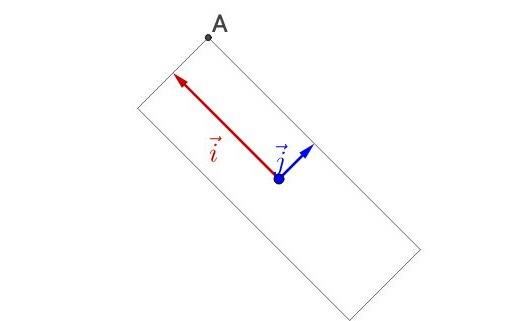$$\begin{array}{l} {\beta _{\rm{1}}}{\rm{ = }}{\alpha _{\rm{1}}}\\ {\beta _{\rm{2}}}{\rm{ = }}{\alpha _2} - - \frac{{{\rm{ < }}{\alpha _2}{\rm{,}}{\beta _{\rm{1}}}{\rm{ > }}}}{{ < {\beta _{\rm{1}}},{\beta _{\rm{1}}} > }}{\beta _{\rm{1}}} \end{array}$$

## 3二次型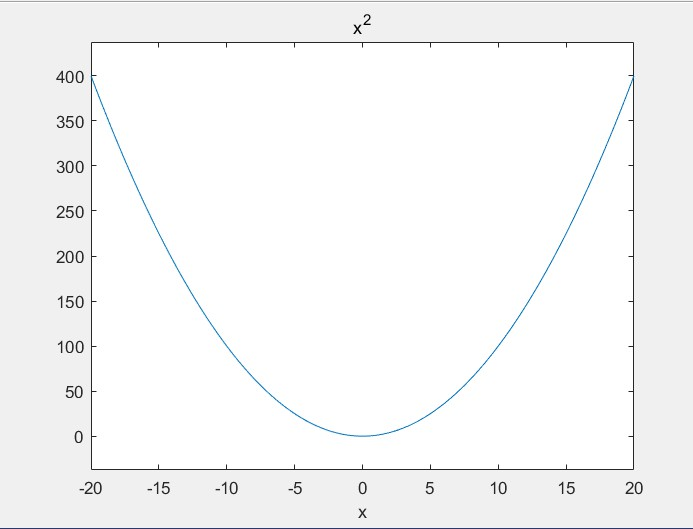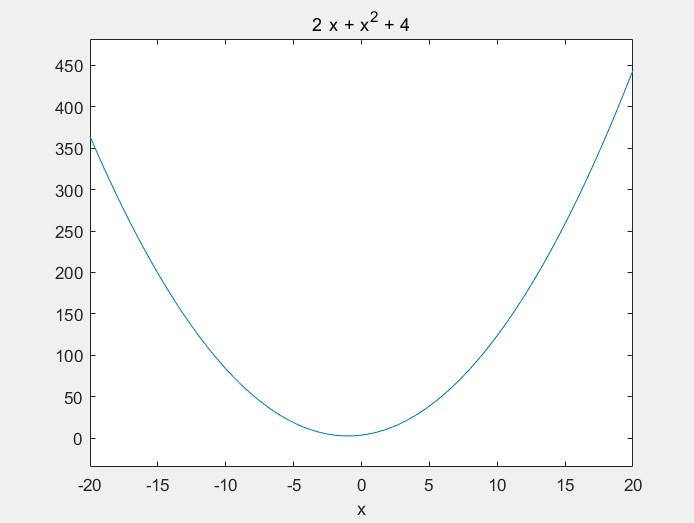### 3.1二次型的平移与旋转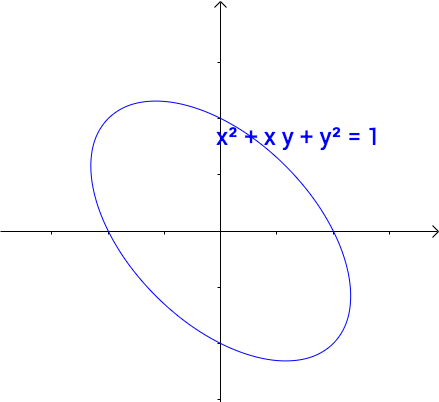$$(x,y)\left[ {\begin{array}{*{20}{c}} {\rm{1}}&{{\rm{0}}{\rm{.5}}}\\ {{\rm{0}}{\rm{.5}}}&{\rm{1}} \end{array}} \right]\left( {\begin{array}{*{20}{c}} x\\ y \end{array}} \right) = 1$$

$$\begin{array}{l} X = {(x,y)^T}\\ A = \left[ {\begin{array}{*{20}{c}} {\rm{1}}&{{\rm{0}}{\rm{.5}}}\\ {{\rm{0}}{\rm{.5}}}&{\rm{1}} \end{array}} \right] \end{array}$$

### 3.2二元二次型的规范化

$$\begin{array}{l} P = \left[ {\begin{array}{*{20}{c}} { - \frac{{\sqrt 2 }}{2}}&{\frac{{\sqrt 2 }}{2}}\\ {\frac{{\sqrt 2 }}{2}}&{\frac{{\sqrt 2 }}{2}} \end{array}} \right]\\ B = \left[ {\begin{array}{*{20}{c}} {0.5}&0\\ 0&{1.5} \end{array}} \right] \end{array}$$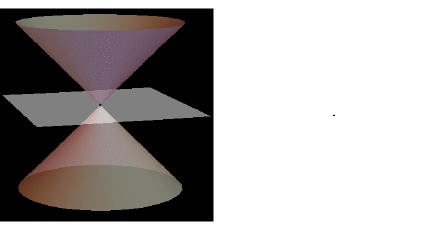### 3.3多元二次型的规范化

1. 列写函数的二次型对称矩阵
2. 计算特征值
3. 将特征值整理成规范化矩阵
4. 将变量分别左乘和右乘规范化矩阵

### 3.4二次型函数的符号性质

• 定义：在多维空间中，使得函数值取正或负的所有坐标轴交叉形成的面的集合为0面。

1. 函数图像均在0面以上，只有个别特殊点函数值等于0，则$f(x)$为正定二次型函数
2. 函数图像均在0面以上，但图像与0面有交线，或有无穷个点使得函数值等于0，则$f(x)$为半正定二次型函数
3. 函数图像均在0面以下，只有个别特殊点函数值等于0，则$f(x)$为负定二次型函数
4. 函数图像均在0面以下，但图像与0面有交线，或有无穷个点使得函数值等于0，则$f(x)$为半负定二次型函数
5. 当函数值在0面任意上下时，称$f(x)$为不定二次型

• 当二次型矩阵特征值均大于0，则$f(x)$为正定二次型函数，二次型矩阵为正定矩阵
• 当二次型矩阵特征值均大于等于0，且至少存在1个0，则$f(x)$为半正定二次型函数，二次型矩阵为半正定矩阵
• 当二次型矩阵特征值均小于0，则$f(x)$为正负定二次型函数，二次型矩阵为负定矩阵
• 当二次型矩阵特征值均小于等于0，且至少存在1个0，则$f(x)$为半负定二次型函数，二次型矩阵为半负定矩阵
• 当二次型矩阵特征值有正有负，则$f(x)$为不定二次型，二次型矩阵为不定矩阵。

## 相关资料

https://www.eatrice.cn/post/线性代数-矩阵与图形/

2020年7月21日